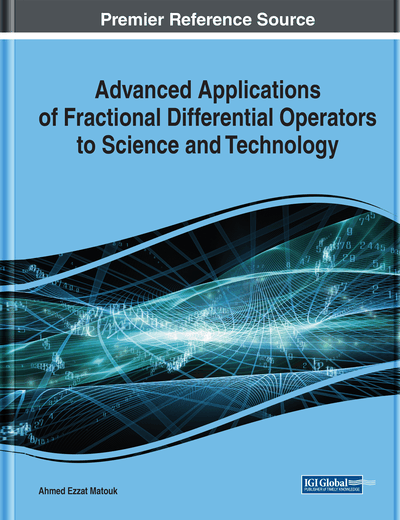# Fractional-Order-Based Inferential Control: A Case Study for the Level Control of Three Interacting Tank Processes

Abdul Wahid Nasir (CMR Institute of Technology, Bengaluru, India), Idamakanti Kasireddy (Vaagdevi College of Engineering, Warangal, India), and Arun Kumar Singh (National Institute of Technology, Jamshedpur, India)
DOI: 10.4018/978-1-7998-3122-8.ch014
Available
\$29.50
No Current Special Offers

## Abstract

This chapter presents the application of fractional differential operator in modelling and control of a three-tank interacting level process. In cases where the usage of sensors for the measurement of primary variable, which is the level of third tank in present case, is physically or economically not feasible, the measurement of secondary variable (i.e., second tank level) is used to determine the level of third tank for control purpose, known as inferential control scheme. The process is modeled and linearized around the operating points, resulting in third order plant, which is approximated to lower order integer and non-integer model. Both conventional integer order PI (IO-PI) & PID (IO-PID) and fractional order PI (FO-PI) & (FO-PID) controllers are implemented for this inferential control. Extensive simulation studies performed using MATLAB validate the supremacy of non-integer order model and controller over integer order model and controller. Genetic algorithm (GA) is being applied for both, firstly for reduced order model approximation and secondly for controller tuning.
Chapter Preview
Top

## Introduction

In Model Based Control as the term itself states, model of the plant is required for designing the control structure. Hence the performance of such model based control techniques depends considerably on the accuracy of plants being modelled. Accurate models will give improved controlled response whereas inaccurate models will degrade it. Some of the well-established control techniques falling in this category are Internal Model Control (IMC), Model Predictive Control (MPC) and Inferential Control etc.

The elementary concept of modelling lies in differential equations. Conventionally the order of differential operators incorporated in these differential equations used to be integer in nature. But in recent past a lot of studies has shown that if the order of these operators is generalized to non-integer (fractional), then capturing the dynamics of plants via models becomes more accurate and realistic (Elwakil, 2010; Efe, 2011; Kumar, 2013; Fahim et al, 2018). Research in the area of fractional order modelling and control has increased manifold in the last couple of decades due to the advent of various numerical techniques to deal with non-integer differential and integral operators (Oldham, 1974; Podlubny 1999). These non-integer order fundamental operators, where ‘α’ is the operator order and ‘a’ & ‘t’ gives the limits of the operator is defined as follows:

Different definitions of this fractional integro-differential is given by various experts namely Riemann-Liouville, Caputo, Grunwald-Lentnikov etc. (Monje et al, 2010). Hence control engineers are also making use of this concept in modelling and control (Monje et al, 2008; Wang et al, 2018; Shalaby, 2019), and are able to get satisfactory results. For carrying out all types of simulation work related to non-integer order plants, FOMCON toolbox (Tepljakov et al, 2011), has been used in this chapter.

A good number of cases have been reported in the literature where fractional order modelling has been exploited for IMC (Mamar & Rachid, 2013; Sondhi & Hote, 2014; Nasir & Singh, 2015) and MPC (Boudjehem & Boudjehem, 2012; Rhouma & Bouani, 2014; Joshi et al, 2014), but it has not been used to its potential for Inferential Control Scheme. Therefore, there is a need to investigate the usage of fractional calculus towards its application in Inferential Control. In this control scheme, the value of primary variable is inferred or estimated using the secondary variable, in situations where the measurement of primary variable is not available. Model of the plants plays a vital role in the estimation process. Accurate model of the plant will provide accurate estimation of primary controlled variable, which in turn will improve the control system performance. So, in present scenario non-integer modelling may be used to enhance controller performance owing to the accurate modelling of the plant. Generally, PI or PID type controller is used in Inferential Control, so additionally this scheme of control bears the potential to take advantage of fractional calculus by extending PI & PID to Fractional Order PI (FO-PI) and Fractional Order PID (FO-PID) respectively. Therefore, it is obvious that the utilization of Fractional Calculus (FC) will enhance the performance of Inferential Control, both from modelling as well as control point of view.

## Complete Chapter List

Search this Book:
Reset